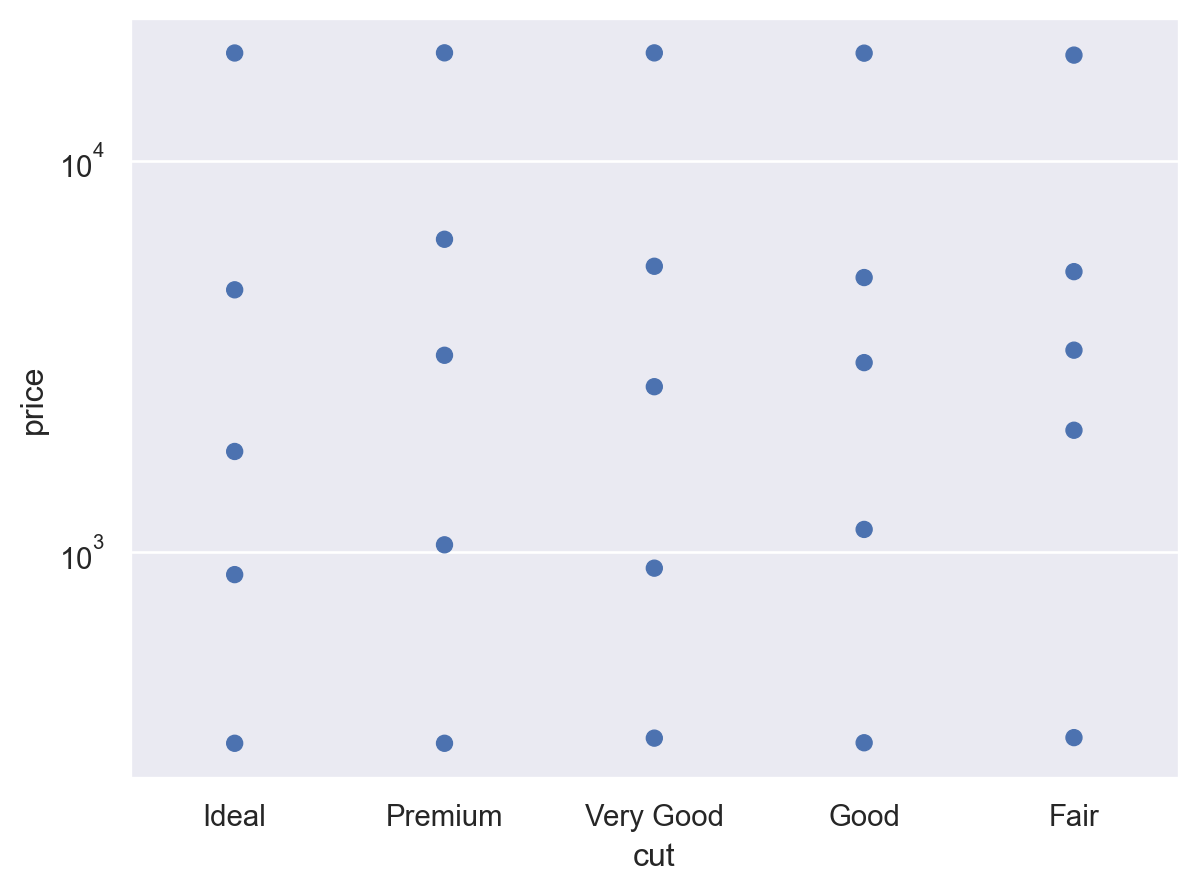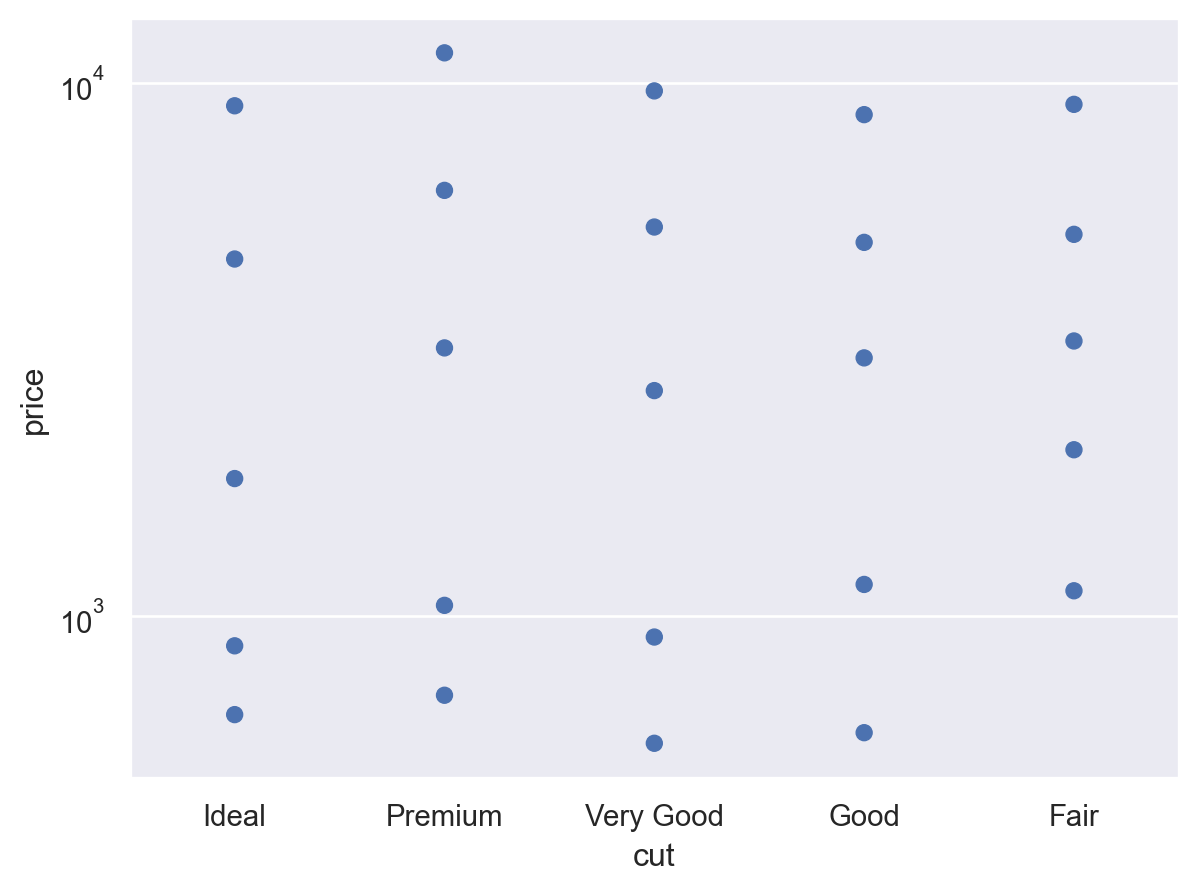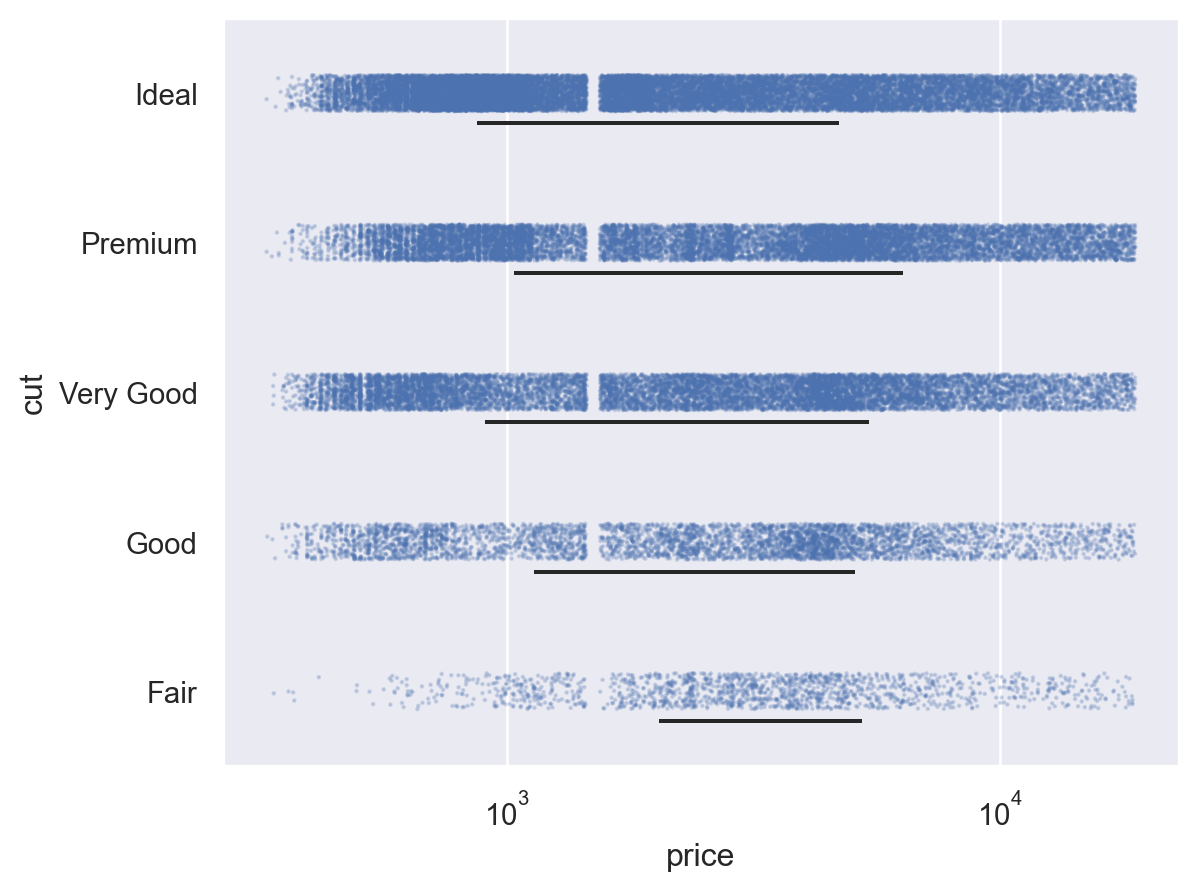# seaborn.objects.Perc#

class seaborn.objects.Perc(k=5, method='linear')#

Replace observations with percentile values.

Parameters:
klist of numbers or int

If a list of numbers, this gives the percentiles (in [0, 100]) to compute. If an integer, compute `k` evenly-spaced percentiles between 0 and 100. For example, `k=5` computes the 0, 25, 50, 75, and 100th percentiles.

methodstr

Method for interpolating percentiles between observed datapoints. See `numpy.percentile()` for valid options and more information.

Examples

The default behavior computes the quartiles and min/max of the input data:

```p = (
so.Plot(diamonds, "cut", "price")
.scale(y="log")
)
```Passing an integer will compute that many evenly-spaced percentiles:

```p.add(so.Dot(), so.Perc(20))
```Passing a list will compute exactly those percentiles:

```p.add(so.Dot(), so.Perc([10, 25, 50, 75, 90]))
```Combine with a range mark to show a percentile interval:

```(
so.Plot(diamonds, "price", "cut")